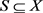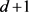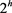﻿ PSL(2,2<sup>n</sup>)与单纯3-(2<sup>n</sup> + 1,2ld + 1,λ)设计(l为奇数，d|(2<sup>n</sup> - 1)且d ≥ 3)

# PSL(2,2n)与单纯3-(2n + 1,2ld + 1,λ)设计(l为奇数，d|(2n - 1)且d ≥ 3)PSL(2,2n) and Simple 3-(2n + 1,2ld + 1,λ) Designs Where l Is Odd, d|(2n - 1) and d ≥ 3

Abstract: Let X=GF(q)∪｛∞｝ be the projective line. Let l be an odd integer. The integer d satisfies d丨（2n-1） and d ≥ 3. In this paper, we determined the parameter set of simple 3-designs from PSL(2,2n) with block size 2ld + 1 where the stabilizer of the initial block contains order d element of PSL(2,2n) and calculated the number of the orbits which form the simple 3-design with that pa-rameter set. By using the orbits of PSL(2,2n) on the X, the results show that the number of the orbits iswhich forms the simple 3-(2n + 1,2ld + 1，2l2ld-1）（2ld+1）） design.

1. 引言

1.1. 研究背景

1) X是一个v-集合；

2) $\mathfrak{B}$ 是X的一组k-子集；

3) X的任意给定的t-子集都恰好含于 $\mathfrak{B}$$\lambda$ 个成员之中。

X的元素称为点， $\mathfrak{B}$ 的成员称为区组。若一个 $t\text{-}\left(v,k,\lambda \right)$ 设计不包含重复的区组，则称这个设计为单纯的。在本文中我们只考虑单纯t-设计。

$G\le sym\left(X\right)$，则对于任意的 $g\in G$${S}^{g}=\left\{{x}^{g}:x\in S\right\}$${S}^{G}=\left\{{S}^{g}:g\in G\right\}$ 称为S的轨道， ${G}_{S}=\left\{g\in G:{S}^{g}=S\right\}$ 称为S的稳定子群，且 $|G|=|{S}^{G}||{G}_{S}|$$\left(X,\mathfrak{B}\right)$ 的一个自同构是指具有下述性质的X的置换g：如果 $B\in \mathfrak{B}$，则 ${B}^{g}\in \mathfrak{B}$。G是 $\left(X,\mathfrak{B}\right)$ 的自同构群当且仅当区组集 $\mathfrak{B}$ 是G作用下X的k-子集的轨道的并。

$LF\left(2,q\right)=\left\{f{|f:X\to X,x}^{f}=\frac{ax+b}{cx+d},ad-bc为非零平方元\right\}$ .

$q={p}^{n}$，其中p为素数。利用 $PSL\left(2,q\right)$ 为设计的自同构群来研究t-设计是一个可行的方法。特别的，文献  已完整解决了以 $PSL\left(2,q\right)$ 为自同构群，区组长度为k的3-设计的存在性问题，其中 $k\overline{)\equiv }0,1\left(\mathrm{mod}p\right)$。文献     完整讨论了以 $PSL\left(2,{2}^{n}\right)$ 为自同构群，区组长度为k ( $4\le k\le 8$ )的单纯3-设计的存在性问题。文献   介绍了以 $PSL\left(2,{2}^{n}\right)$ 为自同构群，区组长度为d和的单纯3-设计的有关结果，这里 $d|\left({2}^{n}-1\right)$。文献  -  找到了一些 $t\ge 4$ 时的t-设计存在的例子。

1.2. 预备知识

1)阶初等Abel群，其中 $h\le n$

2) d阶循环群，其中 $d|\left({2}^{n}\mp 1\right)$

3) 二面体群 ${D}_{2d}$，其中 $d|\left({2}^{n}\mp 1\right)$

4) ${2}^{h}$ 阶的初等Abel群和d阶循环群的半直积，其中 $d|\left({2}^{n}-1\right)$

5) $PSL\left(2,{2}^{k}\right)$，其中 $k|n$

6) 交错群 ${A}_{4}$

2 定理的证明

$S=\left\{1,\alpha ,\cdots ,{\alpha }^{d-1},{\beta }_{1},{\beta }_{1}\alpha ,\cdots ,{\beta }_{1}{\alpha }^{d-1},\cdots ,{\beta }_{m-1},{\beta }_{m-1}\alpha ,\cdots ,{\beta }_{m-1}{\alpha }^{d-1},\infty \right\}$ .

${S}^{\prime }=\left\{1,\alpha ,\cdots ,{\alpha }^{d-1},{{\beta }^{\prime }}_{1},{{\beta }^{\prime }}_{1}\alpha ,\cdots ,{{\beta }^{\prime }}_{1}{\alpha }^{d-1},\cdots ,{{\beta }^{\prime }}_{m-1},{{\beta }^{\prime }}_{m-1}\alpha ,\cdots ,{{\beta }^{\prime }}_{m-1}{\alpha }^{d-1},\infty \right\}$

$\frac{a\alpha x+b\alpha }{cx+d}=\frac{a{\alpha }^{i}x+b}{c{\alpha }^{i}x+d}$ .

$\left[\begin{array}{cc}a\alpha & b\alpha \\ c& d\end{array}\right]=u\left[\begin{array}{cc}a{\alpha }^{i}& b\\ c{\alpha }^{i}& d\end{array}\right]$ .

${S}^{\prime }={S}^{{f}^{\prime }}=\left\{r,r\alpha ,\cdots ,r{\alpha }^{d-1},r{\beta }_{1},r{\beta }_{1}\alpha ,\cdots ,r{\beta }_{1}{\alpha }^{d-1},\cdots ,r{\beta }_{m-1},r{\beta }_{m-1}\alpha ,\cdots ,r{\beta }_{m-1}{\alpha }^{d-1},\infty \right\}$ .

${S}^{\prime }=\left\{1,\alpha ,\cdots ,{\alpha }^{d-1},{{\beta }^{\prime }}_{1},{{\beta }^{\prime }}_{1}\alpha ,\cdots ,{{\beta }^{\prime }}_{1}{\alpha }^{d-1},\cdots ,{{\beta }^{\prime }}_{m-1},{{\beta }^{\prime }}_{m-1}\alpha ,\cdots ,{{\beta }^{\prime }}_{m-1}{\alpha }^{d-1},\infty \right\}$，令 $H=〈\alpha 〉$，从而 $r\in H$$r\in {{\beta }^{\prime }}_{j}H$，其中 $1\le j\le m-1$。下分两种情况讨论：

1) 若 $r\in H$，从而 ${S}^{\prime }=S$

2) 若 $r\in {{\beta }^{\prime }}_{\text{1}}H$，不失一般性，可令 $r\in {{\beta }^{\prime }}_{\text{1}}H$，从而

${S}^{\prime }=\left\{{{\beta }^{\prime }}_{1},{{\beta }^{\prime }}_{1}\alpha ,\cdots ,{{\beta }^{\prime }}_{1}{\alpha }^{d-1},{{\beta }^{\prime }}_{1}{\beta }_{1},{{\beta }^{\prime }}_{1}{\beta }_{1}\alpha ,\cdots ,{{\beta }^{\prime }}_{1}{\beta }_{1}{\alpha }^{d-1},\cdots ,{{\beta }^{\prime }}_{1}{\beta }_{m-1},{{\beta }^{\prime }}_{1}{\beta }_{m-1}\alpha ,\cdots ,{{\beta }^{\prime }}_{1}{\beta }_{m-1}{\alpha }^{d-1},\infty \right\}$ .

${S}^{\prime }=\left\{{\beta }_{k}^{-1},{\beta }_{k}^{-1}\alpha \cdots {\beta }_{k}^{-1}{\alpha }^{d-1},{\beta }_{k}^{-1}{\beta }_{1},{\beta }_{k}^{-1}{\beta }_{1}\alpha ,\cdots ,{\beta }_{k}^{-1}{\beta }_{1}{\alpha }^{d-1},\cdots ,{\beta }_{k}^{-1}{\beta }_{m-1},{\beta }_{k}^{-1}{\beta }_{m-1}\alpha ,\cdots ,{\beta }_{k}^{-1}{\beta }_{m-1}{\alpha }^{d-1},\infty \right\}$ .

${x}^{{g}_{k}}={\beta }_{k}^{-1}x$ Math_207#，其中 ${\beta }_{0}=1$，则上述 ${S}^{\prime }={S}^{{g}_{k}}$。综上述若 ${S}^{\prime }\in {S}^{\mathcal{G}}$ 当且仅当 ${S}^{\prime }={S}^{{g}_{k}}$ Math_212#。

$\left\{{\beta }_{t},{\beta }_{t}\alpha ,\cdots ,{\beta }_{t}{\alpha }^{d-1}\right\}=\left\{{\beta }_{s},{\beta }_{s}\alpha ,\cdots ,{\beta }_{s}{\alpha }^{d-1}\right\}$ .

NOTES

*通讯作者。

 Cameron, P.J., Maimani, H.R., Omidi, G.R. and Tayfeh-Rezaie, B. (2006) 3-Designs from . Discrete Mathematics, 306, 3063-3073.
https://doi.org/10.1016/j.disc.2005.06.041

 Keranen, M.S. and Kreher, D.L. (2004) 3-Designs of with Block Sizes 4 and 5. Journal of Combinarorial Designs, 12, 103-111.
https://doi.org/10.1002/jcd.10068

 Li, W. and Shen, H. (2008) Simple 3-Designs of with Block Size 6. Discrete Mathematics, 308, 3061-3072.
https://doi.org/10.1016/j.disc.2007.08.030

 Li, W. and Shen, H. (2010) Simple 3-Designs of with Block Size 7. Journal of Combinarorial Designs, 16, 1-17.
https://doi.org/10.1002/jcd.20141

 Gong, L.Z. and Fan, G.B. (2018) Simple 3-Designs of with Block Size 8. Utilitas Mathematica, 106, 3-9.

 李伟霞. 区组长度为 因子的单纯3-设计[J]. 上海交通大学学报, 2007, 41(5): 845-847.

 Li, W. and Shen, H. (2011) Simple 3-Designs with Block Sizes from Where . Journal of Combinarorial Designs, 51, 235-241.

 Bierbrauer, J. (1993) A Family of 4-Designs with Block Size 9. Discrete Mathematics, 138, 113-117.
https://doi.org/10.1016/0012-365X(94)00192-L

 刘伟俊, 谭琼华, 龚罗中. 旗传递 设计[J]. 江苏大学学报, 2010, 31(5): 612-615.

 刘伟俊, 姚蹈, 陈静. 一般射影线性群 与 设计[J]. 数学理论与应用, 2010, 30(1): 123-128.

 陈静, 陈暑波, 刘伟俊. 二维射影线性群与区传递 设计[J]. 中国科学, 2010, 40(11): 1045-1054.

 唐剑雄, 陈静, 刘伟俊, 等. 二维射影线性群与区传递 设计[J]. 数学进展, 2012, 41(5): 547-553.

 杨冠, 刘伟俊. 射影线性群区传递作用于 设计[J]. 浙江大学学报, 2013, 40(5): 489-491.

 Dickson, L.E. (1958) Linear Groups with an Introduction to the Galois Field Theory. Dover Publications, New York, 260-287.

Top